# RD Sharma Solutions for Class 9 Maths Chapter 8 Lines and Angles Exercise 8.4

In this exercise, students will be introduced to some new angles made by transversal with two given lines. RD Sharma Class 9 Solutions Chapter 8 Exercise 8.4 also contains answers related to chapters like alternate interior angles, consecutive interior angles and corresponding angles axiom.

## Download PDF of RD Sharma Solutions for Class 9 Maths Chapter 8 Lines and Angles Exercise 8.4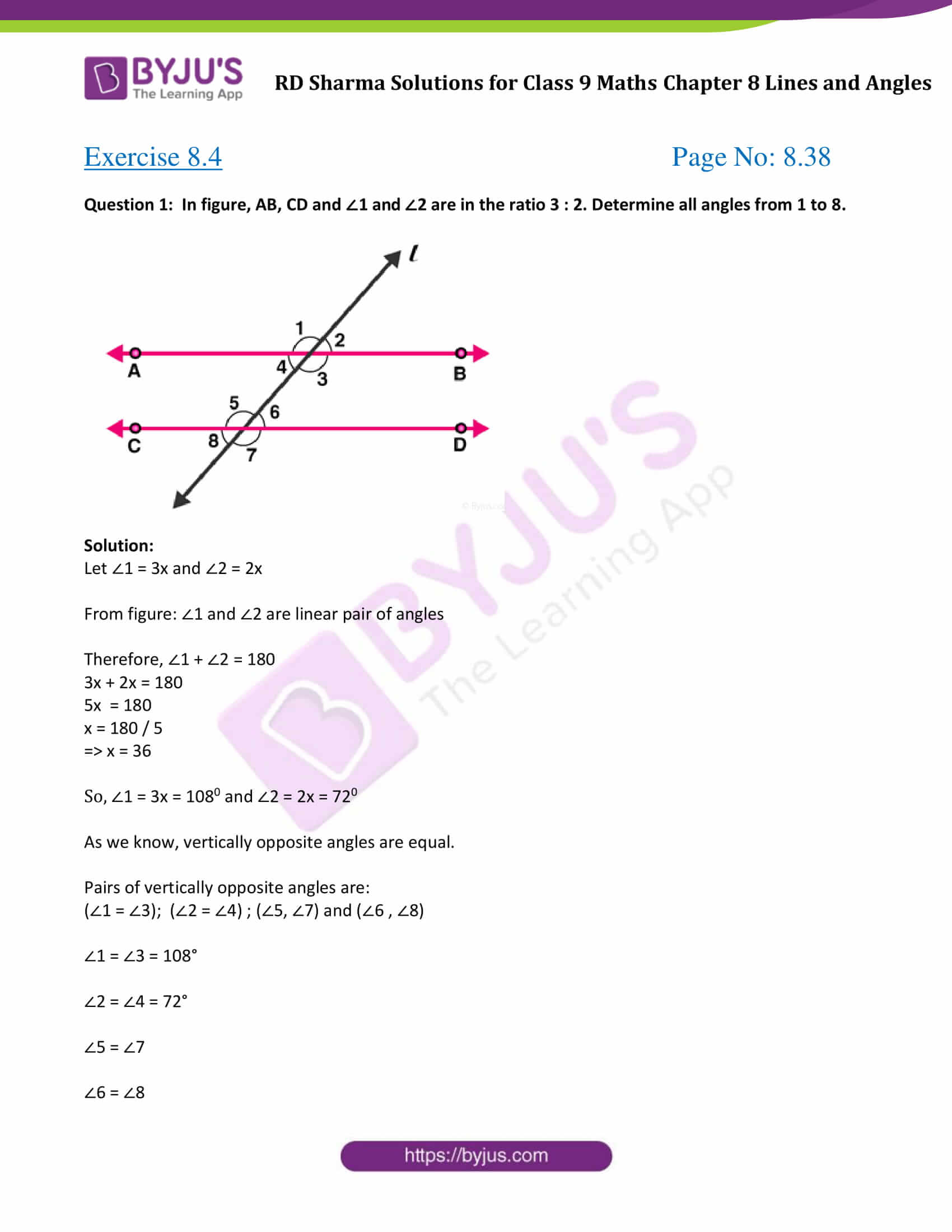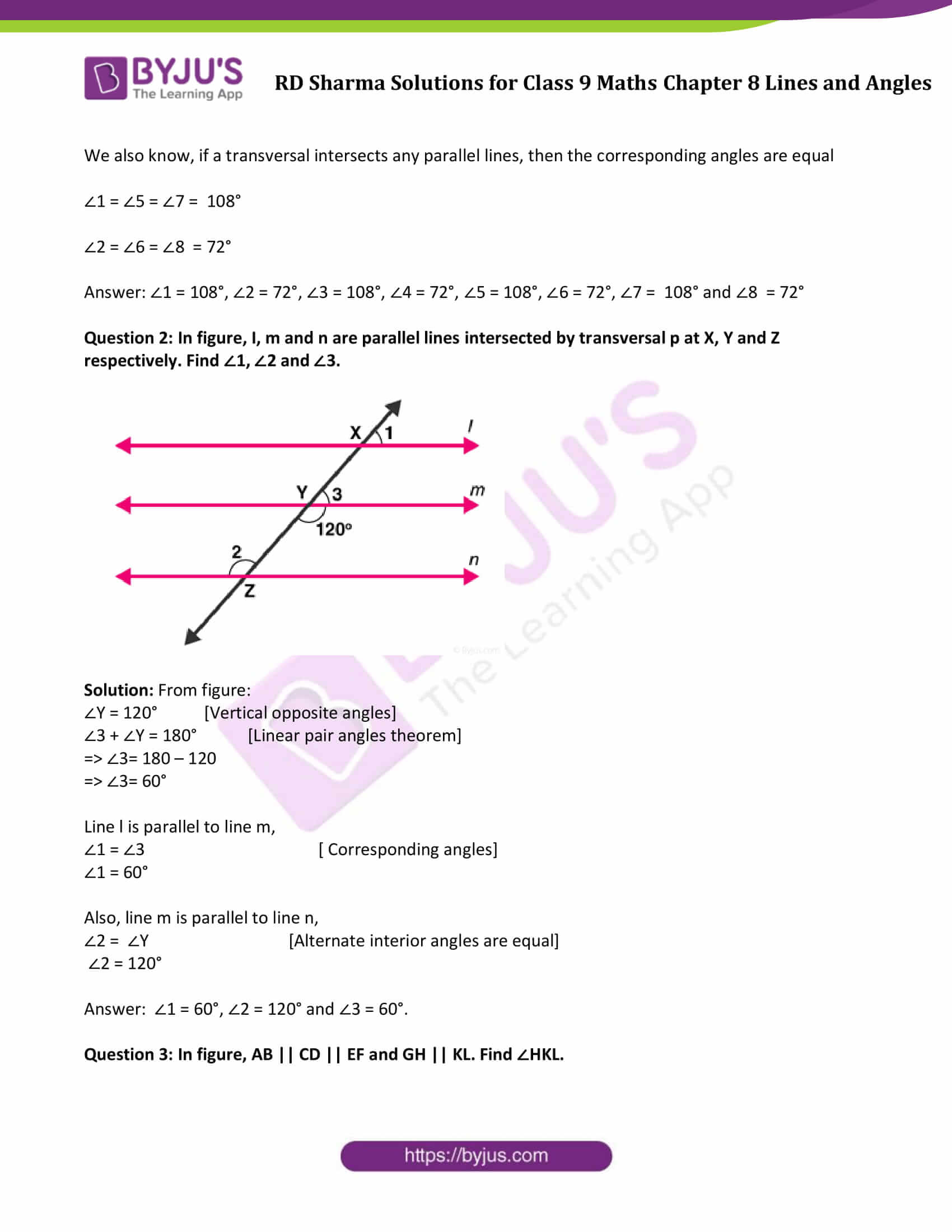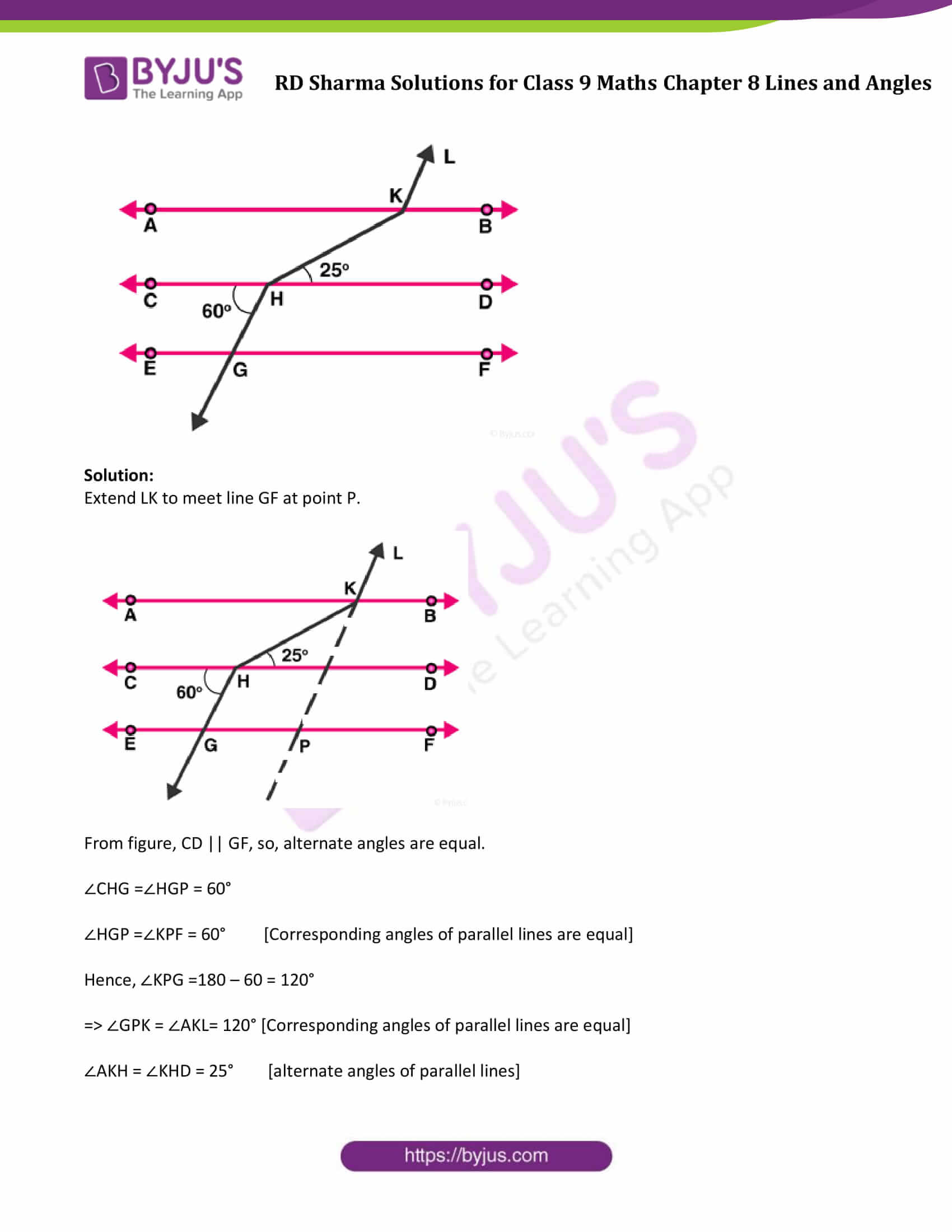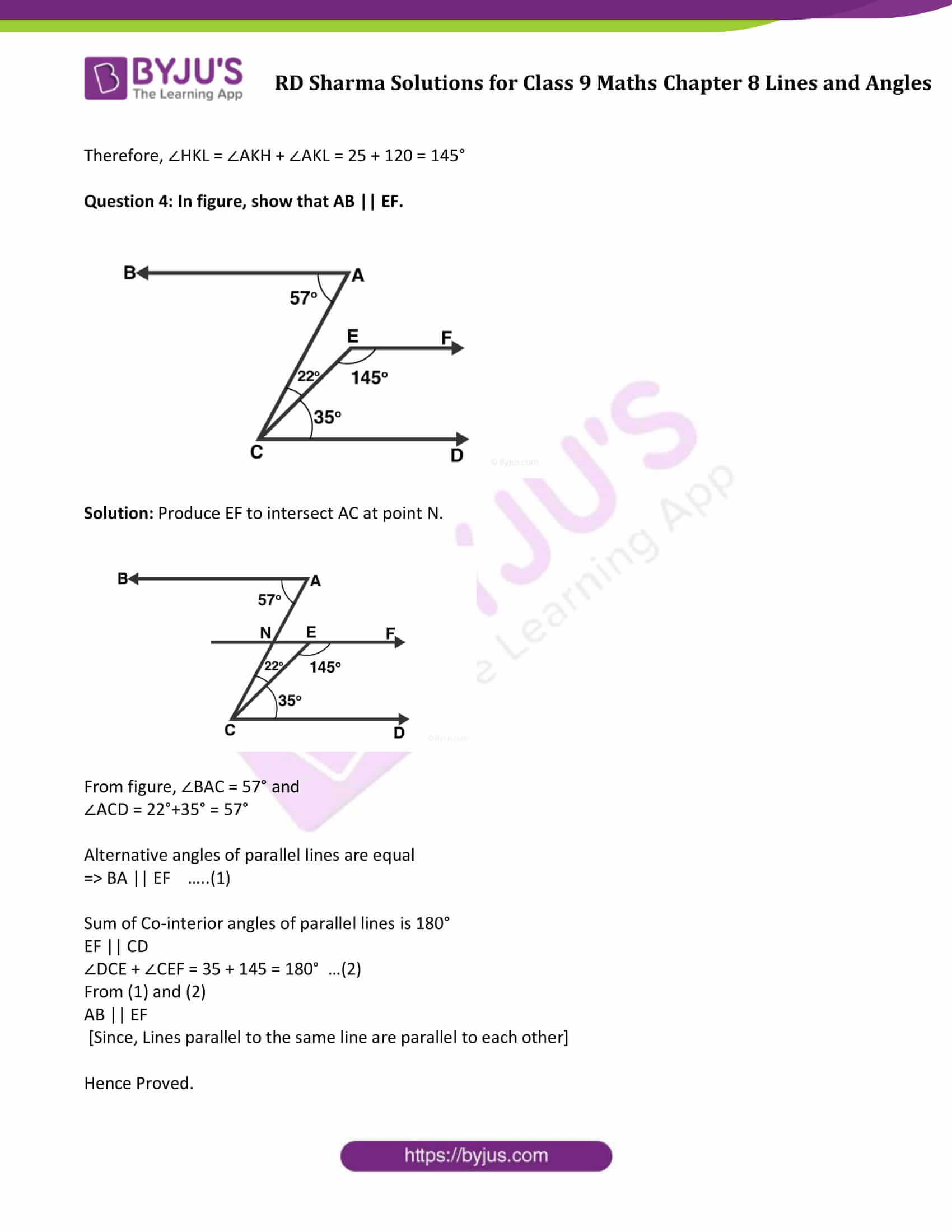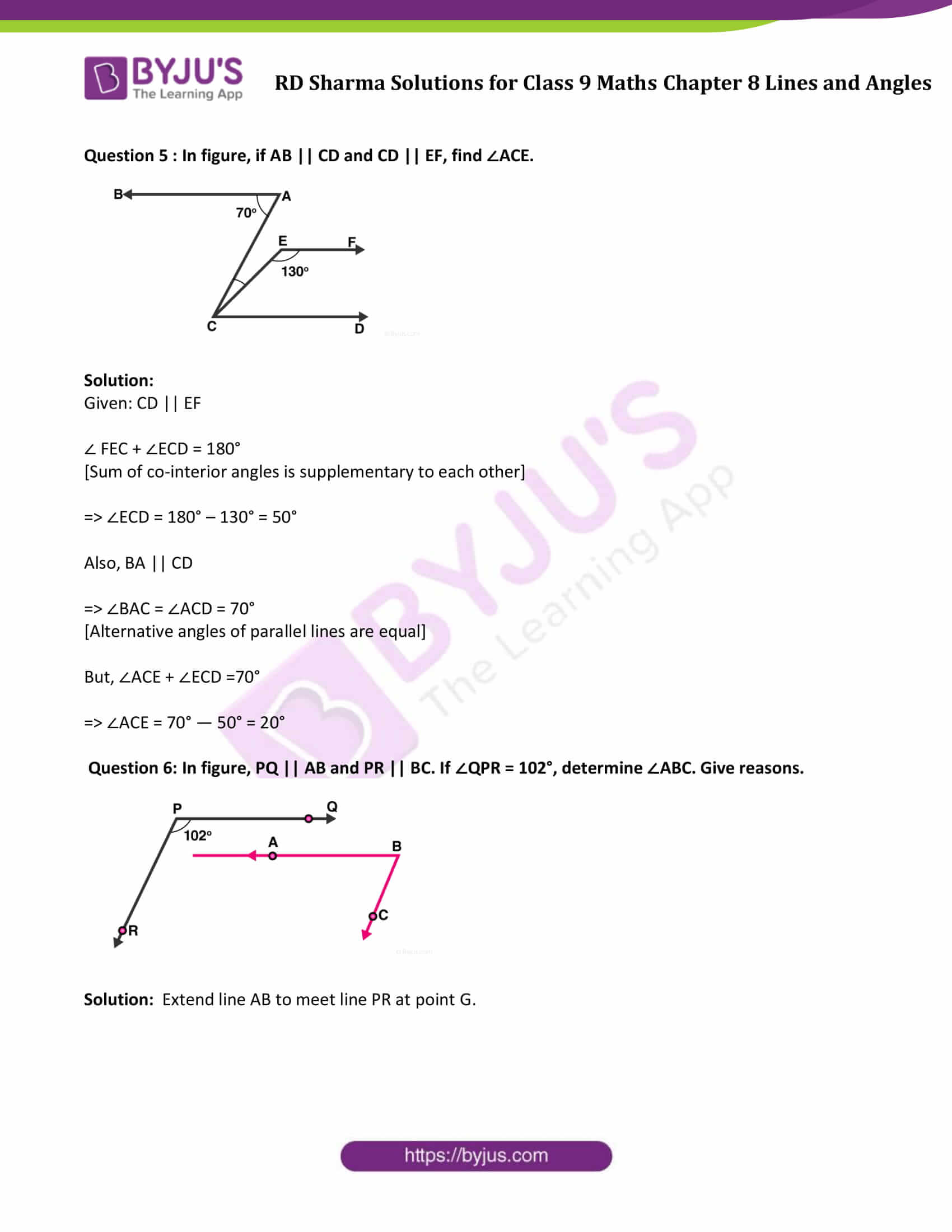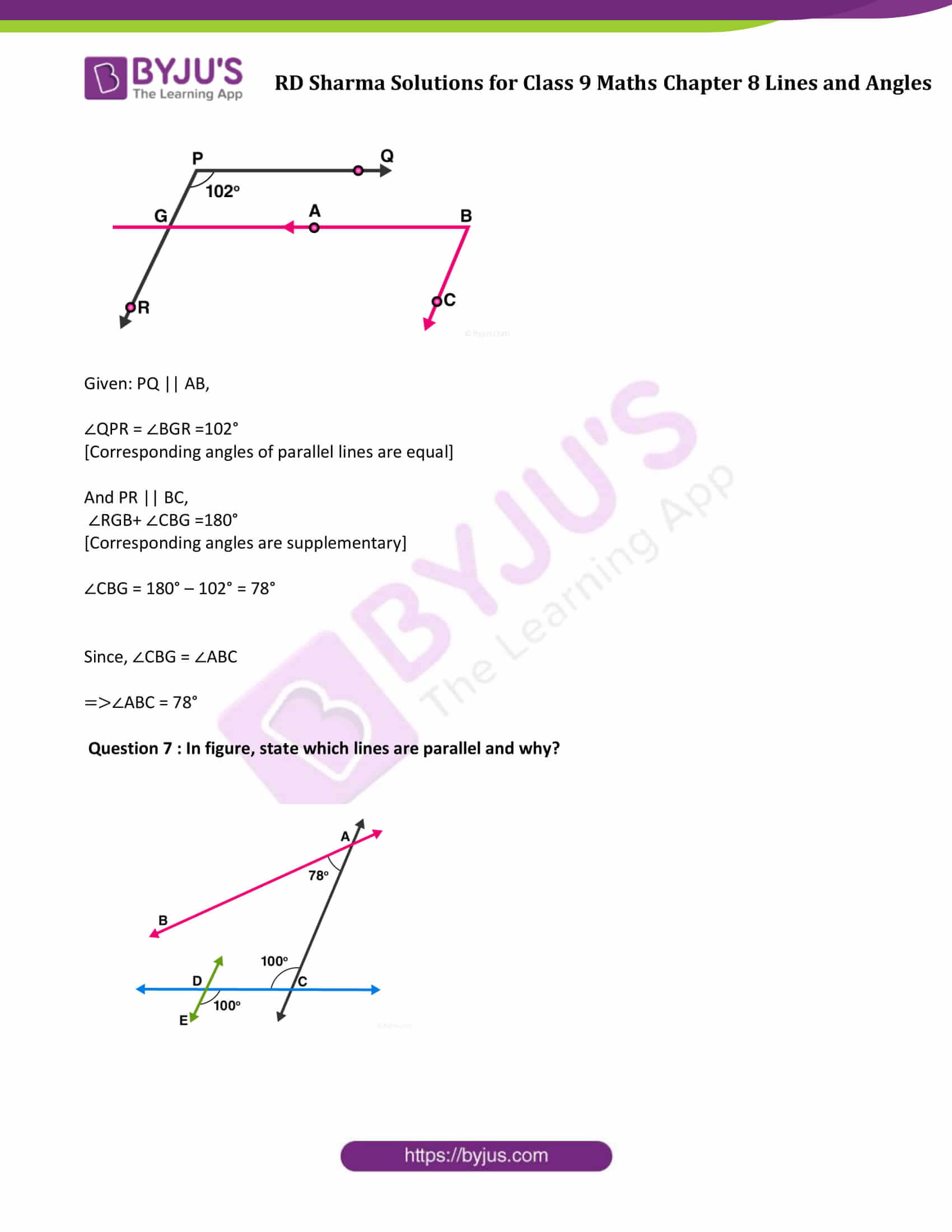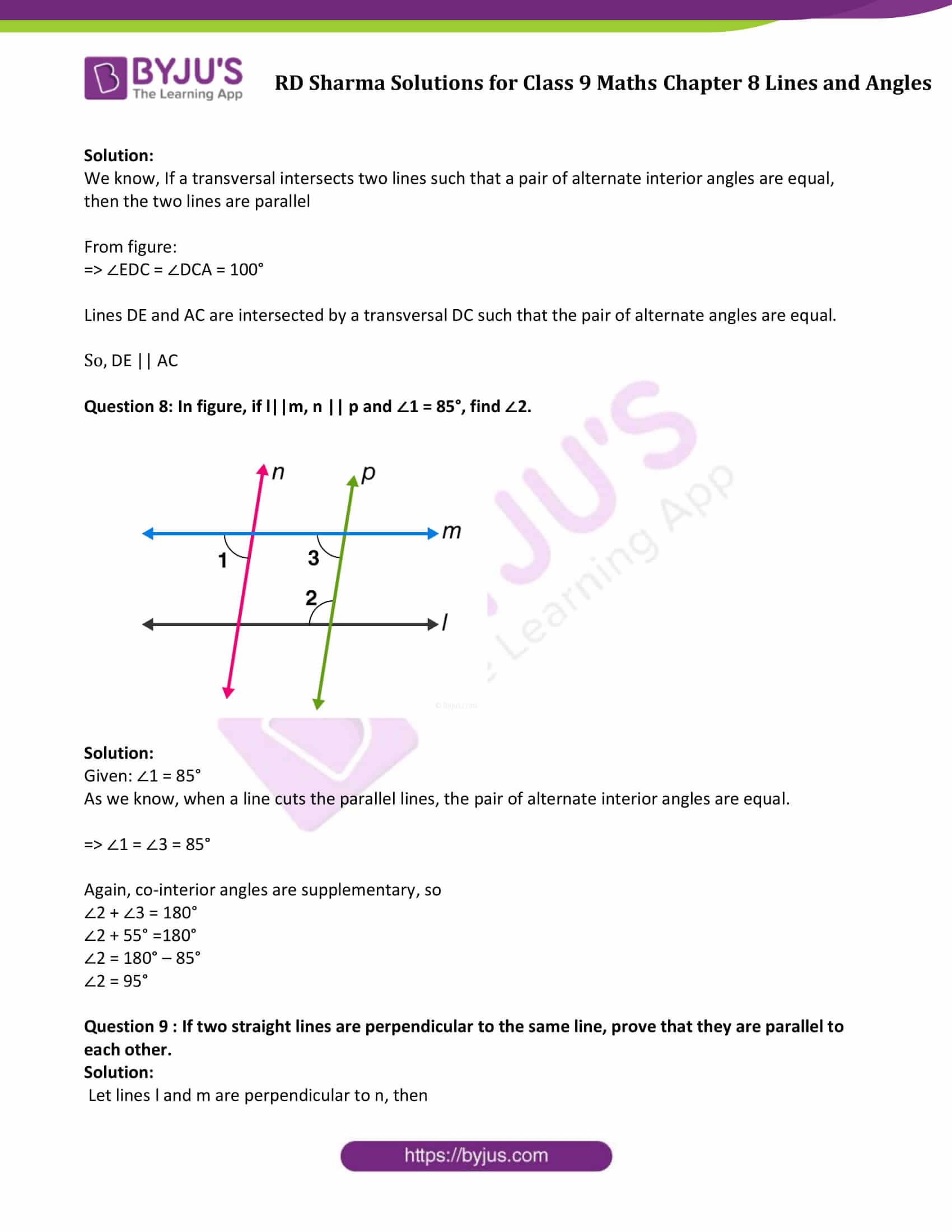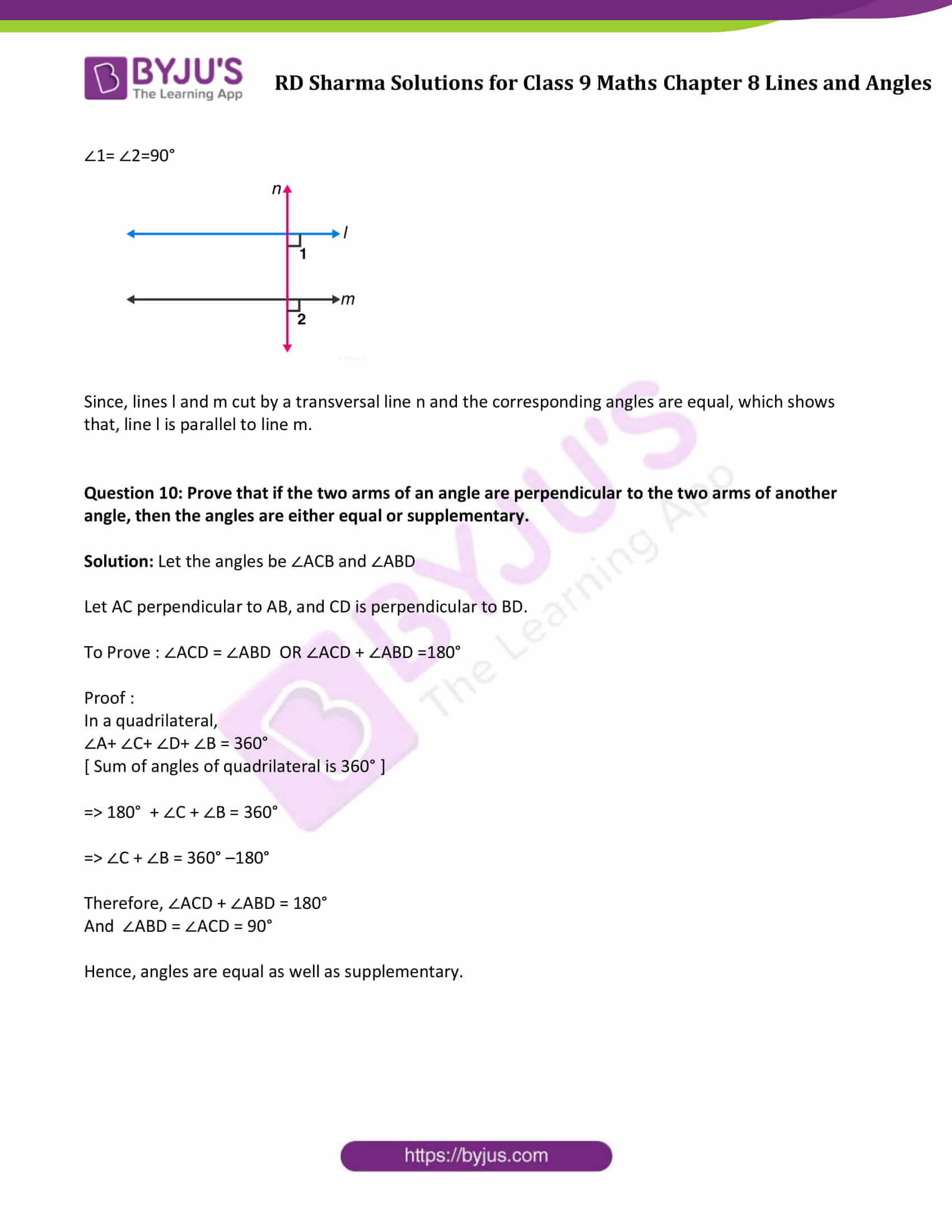### Access Answers to Maths RD Sharma Solutions for Class 9 Chapter 8 Lines and Angles Exercise 8.4 Page number 8.38

Question 1: In figure, AB, CD and ∠1 and ∠2 are in the ratio 3 : 2. Determine all angles from 1 to 8.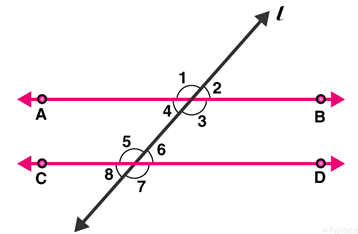Solution:

Let ∠1 = 3x and ∠2 = 2x

From figure: ∠1 and ∠2 are linear pair of angles

Therefore, ∠1 + ∠2 = 180

3x + 2x = 180

5x = 180

x = 180 / 5

=> x = 36

So, ∠1 = 3x = 1080 and ∠2 = 2x = 720

As we know, vertically opposite angles are equal.

Pairs of vertically opposite angles are:

(∠1 = ∠3); (∠2 = ∠4) ; (∠5, ∠7) and (∠6 , ∠8)

∠1 = ∠3 = 108°

∠2 = ∠4 = 72°

∠5 = ∠7

∠6 = ∠8

We also know, if a transversal intersects any parallel lines, then the corresponding angles are equal

∠1 = ∠5 = ∠7 = 108°

∠2 = ∠6 = ∠8 = 72°

Answer: ∠1 = 108°, ∠2 = 72°, ∠3 = 108°, ∠4 = 72°, ∠5 = 108°, ∠6 = 72°, ∠7 = 108° and ∠8 = 72°

Question 2: In figure, I, m and n are parallel lines intersected by transversal p at X, Y and Z respectively. Find ∠1, ∠2 and ∠3.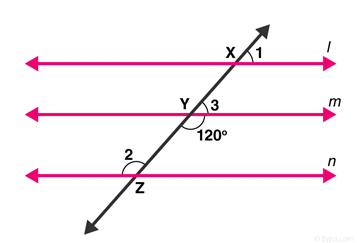Solution: From figure:

∠Y = 120° [Vertical opposite angles]

∠3 + ∠Y = 180° [Linear pair angles theorem]

=> ∠3= 180 – 120

=> ∠3= 60°

Line l is parallel to line m,

∠1 = ∠3 [ Corresponding angles]

∠1 = 60°

Also, line m is parallel to line n,

∠2 = ∠Y [Alternate interior angles are equal]

∠2 = 120°

Answer: ∠1 = 60°, ∠2 = 120° and ∠3 = 60°.

Question 3: In figure, AB || CD || EF and GH || KL. Find ∠HKL.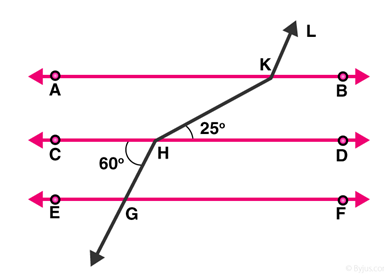Solution:

Extend LK to meet line GF at point P.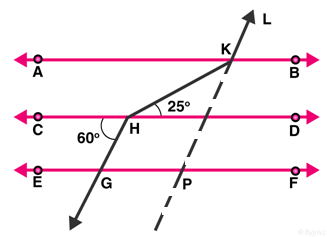From figure, CD || GF, so, alternate angles are equal.

∠CHG =∠HGP = 60°

∠HGP =∠KPF = 60° [Corresponding angles of parallel lines are equal]

Hence, ∠KPG =180 – 60 = 120°

=> ∠GPK = ∠AKL= 120° [Corresponding angles of parallel lines are equal]

∠AKH = ∠KHD = 25° [alternate angles of parallel lines]

Therefore, ∠HKL = ∠AKH + ∠AKL = 25 + 120 = 145°

Question 4: In figure, show that AB || EF.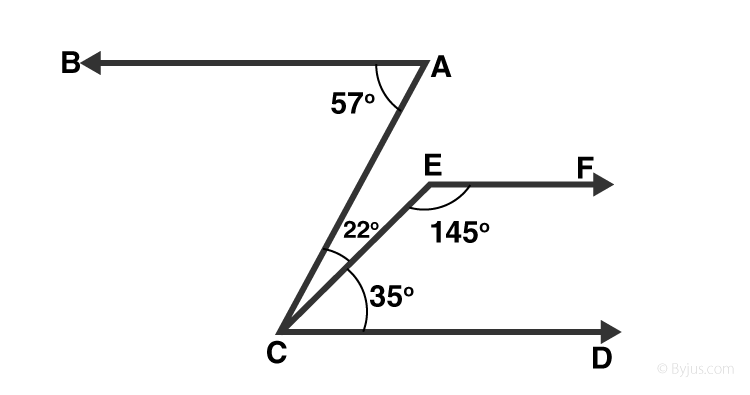Solution: Produce EF to intersect AC at point N.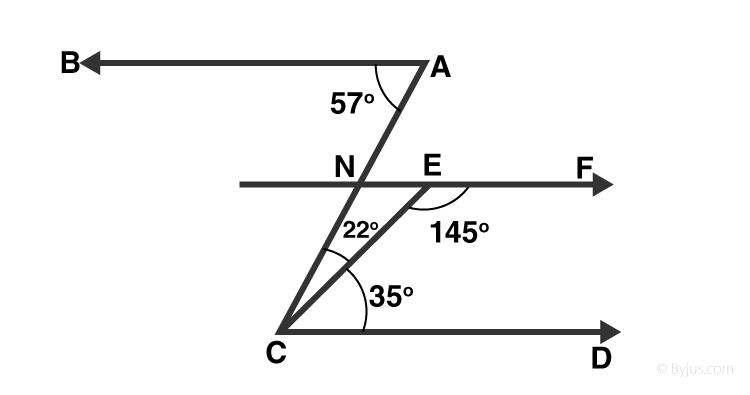From figure, ∠BAC = 57° and

∠ACD = 22°+35° = 57°

Alternative angles of parallel lines are equal

=> BA || EF …..(1)

Sum of Co-interior angles of parallel lines is 180°

EF || CD

∠DCE + ∠CEF = 35 + 145 = 180° …(2)

From (1) and (2)

AB || EF

[Since, Lines parallel to the same line are parallel to each other]

Hence Proved.

Question 5 : In figure, if AB || CD and CD || EF, find ∠ACE.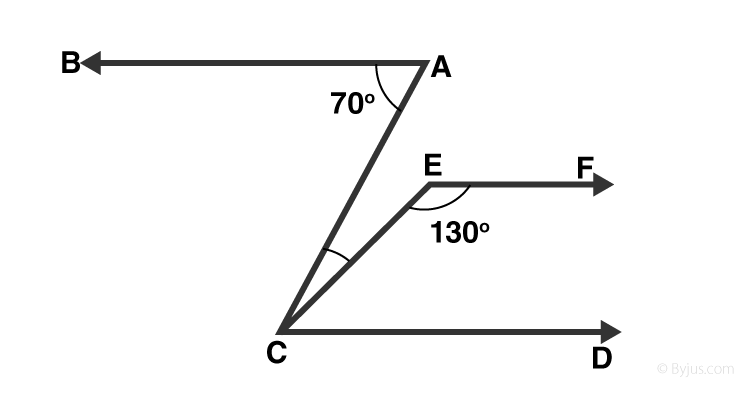Solution:

Given: CD || EF

∠ FEC + ∠ECD = 180°

[Sum of co-interior angles is supplementary to each other]

=> ∠ECD = 180° – 130° = 50°

Also, BA || CD

=> ∠BAC = ∠ACD = 70°

[Alternative angles of parallel lines are equal]

But, ∠ACE + ∠ECD =70°

=> ∠ACE = 70° — 50° = 20°

Question 6: In figure, PQ || AB and PR || BC. If ∠QPR = 102°, determine ∠ABC. Give reasons.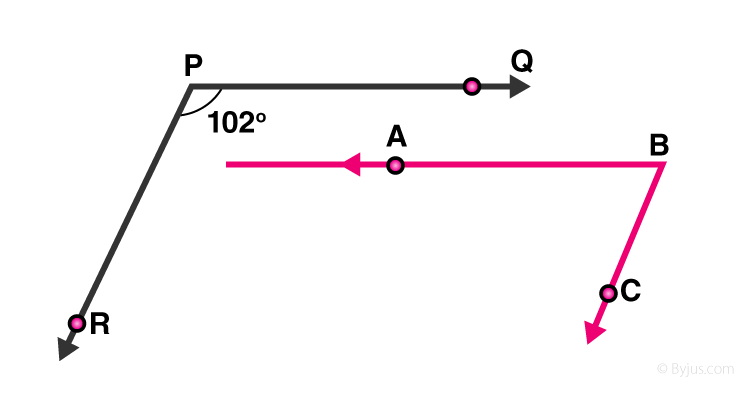Solution: Extend line AB to meet line PR at point G.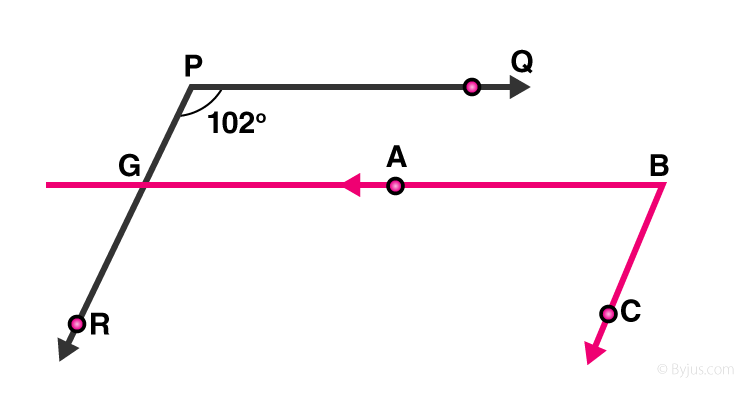Given: PQ || AB,

∠QPR = ∠BGR =102°

[Corresponding angles of parallel lines are equal]

And PR || BC,

∠RGB+ ∠CBG =180°

[Corresponding angles are supplementary]

∠CBG = 180° – 102° = 78°

Since, ∠CBG = ∠ABC

=>∠ABC = 78°

Question 7 : In figure, state which lines are parallel and why?Solution:

We know, If a transversal intersects two lines such that a pair of alternate interior angles are equal, then the two lines are parallel

From figure:

=> ∠EDC = ∠DCA = 100°

Lines DE and AC are intersected by a transversal DC such that the pair of alternate angles are equal.

So, DE || AC

Question 8: In figure, if l||m, n || p and ∠1 = 85°, find ∠2.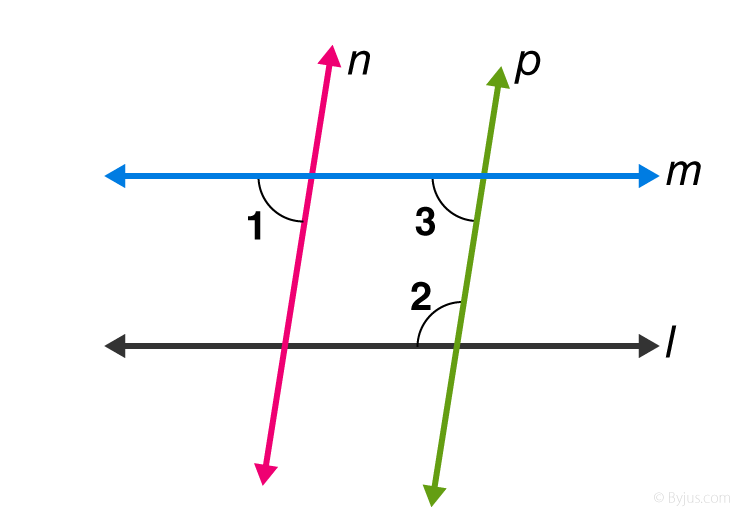Solution:

Given: ∠1 = 85°

As we know, when a line cuts the parallel lines, the pair of alternate interior angles are equal.

=> ∠1 = ∠3 = 85°

Again, co-interior angles are supplementary, so

∠2 + ∠3 = 180°

∠2 + 55° =180°

∠2 = 180° – 85°

∠2 = 95°

Question 9 : If two straight lines are perpendicular to the same line, prove that they are parallel to each other.

Solution:

Let lines l and m are perpendicular to n, then

∠1= ∠2=90°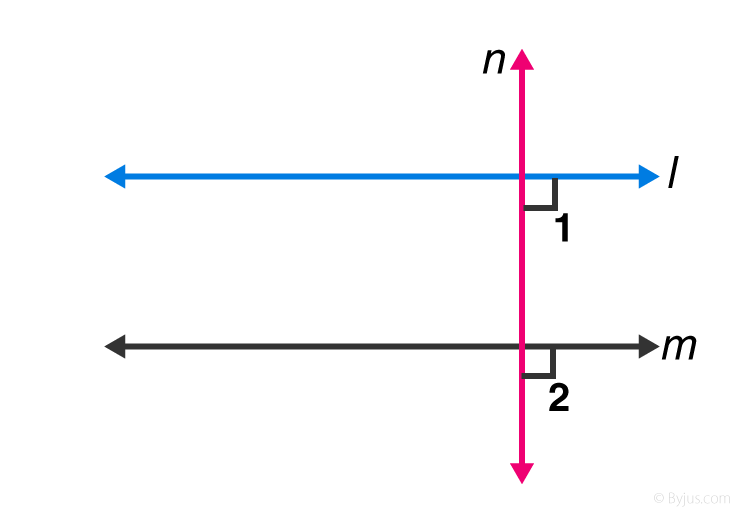Since, lines l and m cut by a transversal line n and the corresponding angles are equal, which shows that, line l is parallel to line m.

Question 10: Prove that if the two arms of an angle are perpendicular to the two arms of another angle, then the angles are either equal or supplementary.

Solution: Let the angles be ∠ACB and ∠ABD

Let AC perpendicular to AB, and CD is perpendicular to BD.

To Prove : ∠ACD = ∠ABD OR ∠ACD + ∠ABD =180°

Proof :

∠A+ ∠C+ ∠D+ ∠B = 360°

[ Sum of angles of quadrilateral is 360° ]

=> 180° + ∠C + ∠B = 360°

=> ∠C + ∠B = 360° –180°

Therefore, ∠ACD + ∠ABD = 180°

And ∠ABD = ∠ACD = 90°

Hence, angles are equal as well as supplementary.

## RD Sharma Solutions for Class 9 Maths Chapter 8 Lines and Angles Exercise 8.4

RD Sharma Solutions Class 9 Maths Chapter 8 Lines and Angles Exercise 8.4 is based on the following topics and subtopics:

• Angles made by a transversal with two lines
• Alternate interior angles
• Consecutive interior angles
• Corresponding angles axiom